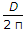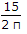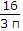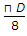# Civil Engineering - UPSC Civil Service Exam Questions

36.

A reinforced concrete beam is designed for the limit states of collapse in flexure a shear. Which of the following limit states of serviceability have to be checked ?
1. Deflection
2. Cracking
3. Durability
Select the correct answer using the codes given below :

 A. 1 alone B. 1 and 2 C. 2 and 3 D. 1, 2 and 3

Explanation:

No answer description available for this question. Let us discuss.

37.

Two men, one stronger than the other have to lift a load of 1200 N which is suspended from a light rod of length 3 m. The load is suspended between the two persons positioned at the two ends of the rod. The weaker of the two persons can carry a load up to 400 N only. The distance of the load to be suspended from the stronger person such that the weaker person has the full share of 400 N is

 A. 0.5 m B. 1.0 m C. 1.5 m D. 2.0 m

Explanation:

No answer description available for this question. Let us discuss.

38.

In a closed theodolite traverse, the sum of the latitudes is + 5.080 m and the sum of the departures is -51.406 m. The sum of the traverse legs is 20.525 km. The accuracy of traverse is nearly equal to

 A. 1 : 300 B. 1 : 400 C. 1 : 500 D. 1 : 1000

Explanation:

No answer description available for this question. Let us discuss.

39.

Consider the following statements :
1. Pumps in series operation allow the head to increase.
2. Pumps in series operation increase the flow rate.
3. Pumps ill parallel operation increase the flow rate.
4. Pumps in parallel operation allow the head to increase.
Which of these statements are correct ?

 A. 1 and 3 B. 1 and 4 C. 2 and 4 D. 3 and 4

Explanation:

No answer description available for this question. Let us discuss.

40.

The shape factor for a solid circular section of diameter D is equal to

 A.B.C.D.